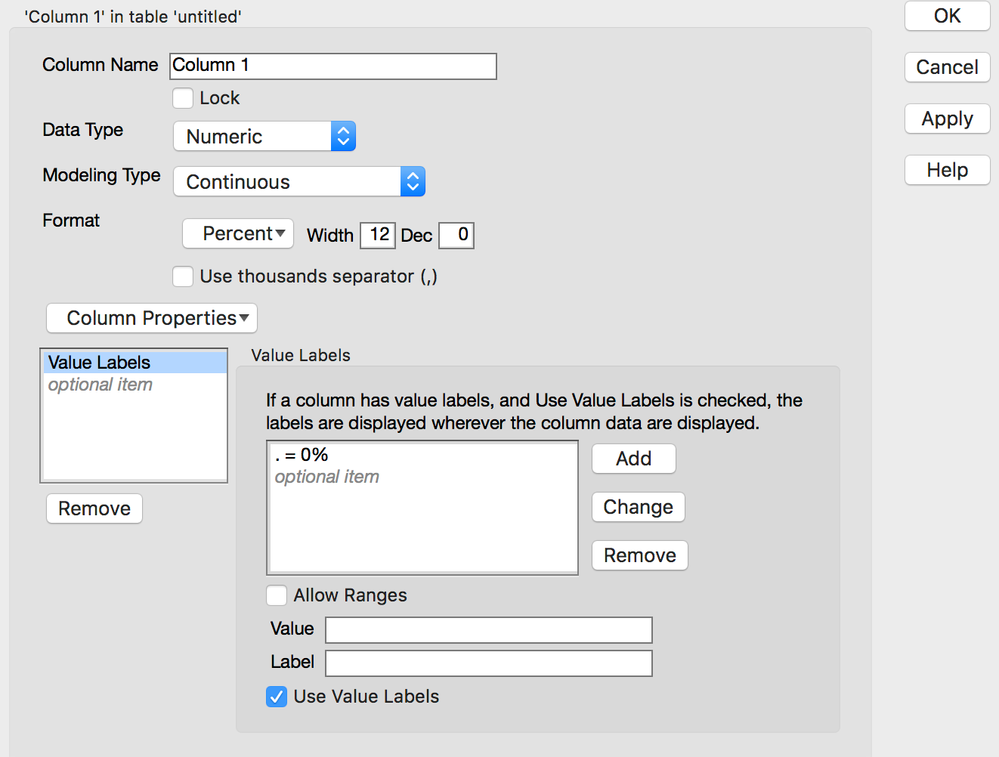Choose Language Hide Translation Bar
Highlighted

## using Percentage Formula but i want it to show as 0.0% instead of "." or missing data only

I'm using percentage formula but the formula shows "." only when i divide 0 to 0. (0/0)

how can it show 0.0% instead?

thanks

2 REPLIES 2
Highlighted

## Re: using Percentage Formula but i want it to show as 0.0% instead of "." or missing data only

Use a conditional statement in your formula and set the missing value to a zero

``````x = 0 / 0;
If( Is Missing( x ), x = 0 );``````
Jim
Highlighted

## Re: using Percentage Formula but i want it to show as 0.0% instead of "." or missing data only

Alternatively, you could use the 'Value Label' column property:Article Labels

There are no labels assigned to this post.